# Bicycle Model

The non-linear bicycle model considers longitudinal (x), lateral (y), and yaw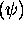motion under the assumption of negligible lateral weight shift, roll and compliance steer while traveling on a smooth road. Our design of control strategy is to control both longitudinal and lateral motions during hard braking and steering maneuvers. Angular velocities of front and rear tires are added to the states in order to investigate directional interactions between longitudinal and lateral tire forces. In addition to these five states, longitudinal and lateral positions and yaw angle with respect to the fixed inertial coordinates are added to the dynamic equation in order to refresh the vehicle position and orientation in the simulation scene. Thus, the bicycle model used in our simulator has 5 Degrees Of Freedom with 8 state equations. The bicycle model developement presented here is based on reference . Figure 1 shows side and top views of the vehicle using this bicycle model. Using free body diagram shown in top view of Figure 1, the equations of motion are derived.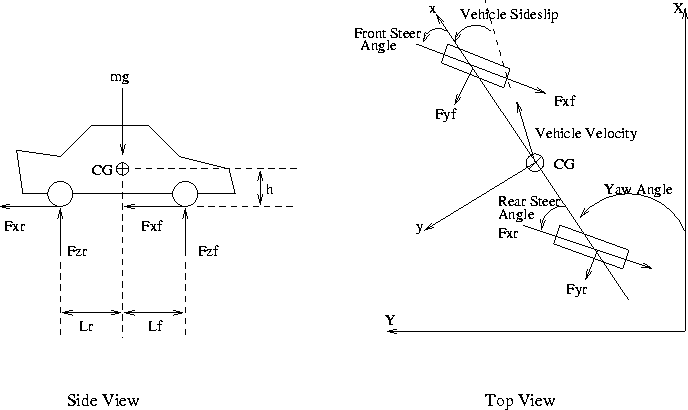Figure 1: Free Body Diagram of a Vehicle

Summing the longitudinal forces along the body x axis leads to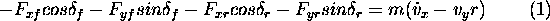Where m is the mass of a vehicle,and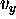are the longitudinal and lateral components of the vehicle velocity resolved along the body axis, r is the yaw rate, and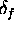and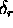are the front and rear wheel steering angles. Summing the lateral forces along the body y axis gives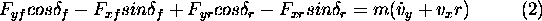The sum of the yaw moments about the car CG yields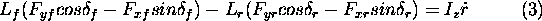Figure 2: Free Body Diagram of a Wheel

For the front and rear wheels, the sum of the torque about the axle, as shown in Figure 2, results in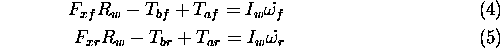Where,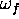and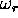are the angular velocities of the front and rear wheels,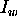is the inertia of the wheel about the axle,is the wheel radius,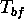andare the applied braking torques, and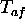and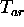are the applied throttling torques for the front and rear wheels. All the vehicle specifications are based on the 1984 Honda Accord  with reasonable braking torques for front and rear tires. Yaw angle is directly found by integrating the yaw rate. Since yaw angle is with respect to the fixed coordinates, longitudinal and lateral position with respect to the inertial fixed coordinates are also found as follows.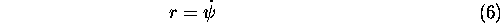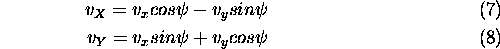Where,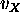and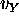denote the velocity components with respect to the fixed inertial coordinates. Simple integration based on the forth-order Runge-Kutta method is used to integrate the above eight states in the simulation loop.

# Tire Force Calculation

The longitudinal and lateral forces from front and rear tires are derived from the non-linear tire model discussed earlier. The input variables for the tire model are front and rear normal loads (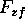and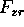), slip angles (and), and longitudinal slip ratios (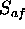and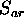). The normal forces of front and rear tires are determined according to the instantaneous longitudinal acceleration. Summing the moments about the rear contact patch using the side view of Figure 1, normal load of front tire is found as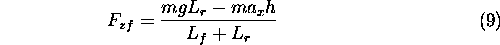Summing the moments about the front contact patch,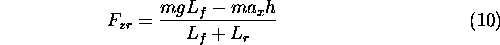Whereis the instantaneous longitudinal acceleration and h is the height of the car CG from the ground.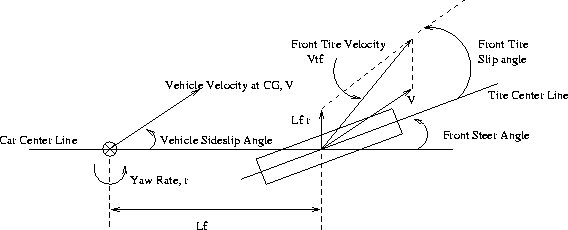Figure 3: Slip Angle of Front WheelFigure 4: Slip Angle of Rear Wheel

From Figure 3 and Figure 4, velocities of front and rear tires are determined by summing the velocity at CG and the velocities effected by the yaw rate. Thus, the slip angles of front and rear tires are found as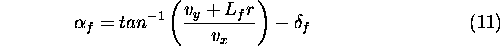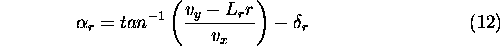Also, the speed of the front and rear tires are calculated by the following equations.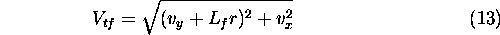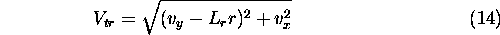Where,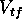and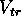represent the magnitude of the front and rear tire axle velocities. To calculate the longitudinal slip, longitudinal component of the tire velocity should be derived. The front and rear longitudinal velocity components are found byThen, the longitudinal slip is determined according to the equation in tire model. Under braking conditions, longitudinal slip of front and rear tires are calculated by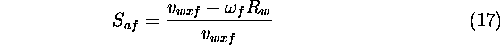Using the normal load, slip angle, longitudinal slip, and non-linear tire model realistic longitudinal and lateral forces are generated for the 5 DOF bicycle model.

## References

1
Taheri, S., An Investigation and Design of Slip Control Braking Systems Integrated with Four Wheel Steering, Ph.D. Thesis, Clemson University, 1990.
2
American Honda Motor Company Inc., Motor Vehicle Specifications, Passenger Car, Accord 1984, Technical Report, Honda, Windsor Locks, 1984.